Difference between revisions of "Cole equation of state"

The Cole equation of state  can be written, when atmospheric pressure is negligible, has the form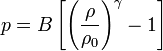$p = B \left[ \left( \frac{\rho}{\rho_0} \right)^\gamma -1 \right]$

In it,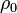$\rho_0$ is a reference density around which the density varies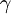$\gamma$ is an exponent and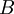$B$ is a pressure parameter.

Usually, the equation is used to model a nearly incompressible system. In this case, the exponent is often set to a value of 7, and$B$ is large, in the following sense. The fluctuations of the density are related to the speed of sound as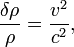$\frac{\delta \rho}{\rho} = \frac{v^2}{c^2} ,$

where$v$ is the largest velocity, and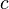$c$ is the speed of sound (the ratio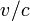$v/c$ is Mach's number). The speed of sound can be seen to be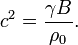$c^2 = \frac{\gamma B}{\rho_0}.$

Therefore, if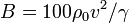$B=100 \rho_0 v^2 / \gamma$, the relative density fluctuations will be of about 0.01.

If the fluctuations in the density are indeed small, the equation of state may be rewritten thus: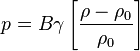$p = B \gamma \left[ \frac{\rho-\rho_0}{\rho_0} \right]$